﻿ 基于NES减振与双向隔振器能量采集一体化分析

# 基于NES减振与双向隔振器能量采集一体化分析Integrated Analysis of NES Vibration Reduction and Dual-Purpose Isolator Energy Harvesting

Abstract: In this paper, a new model of nonlinear energy sink (NES) coupled with dual-purpose isolator is proposed, and its analytical solution and numerical solution are solved by harmonic balance-arc extension method and fourth-order Runge-Kutta method verification analysis. By comparing the amplitude-frequency response of the main system, it is proved that the coupled system has excel-lent vibration-damping performance. The relationship between system displacement velocity and flux density is studied, and the influence of system parameters on voltage and energy is analyzed. The results show that the coupled system can achieve energy harvesting. Further comparison and analysis of the energy dissipation performance of the new coupling system and the traditional NES system, the results show that the coupled system has a higher energy dissipation rate.

1. 引言

2. 模型建立

${m}_{1}{\stackrel{¨}{x}}_{1}+\left({c}_{\text{a}}+{c}_{\text{m}}\right)\left({\stackrel{˙}{x}}_{1}-{\stackrel{˙}{x}}_{2}\right)+\left({k}_{\text{s}}+{k}_{\text{m1}}\right)\left({x}_{1}-{x}_{2}\right)+{k}_{\text{m3}}{\left({x}_{1}-{x}_{2}\right)}^{3}=0$ (1)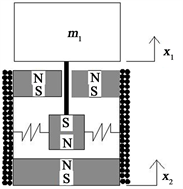(a)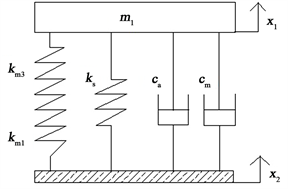(b)

Figure 1. Dual-purpose vibration isolator schematic (a) and mechanical model diagram (b)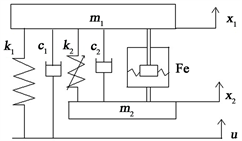Figure 2. System model

$\left\{\begin{array}{l}{m}_{1}{\stackrel{¨}{x}}_{1}+{k}_{1}\left({x}_{1}-u\right)+{c}_{1}\left({\stackrel{˙}{x}}_{1}-\stackrel{˙}{u}\right)+{k}_{2}{\left({x}_{1}-{x}_{2}\right)}^{3}+{c}_{2}\left({\stackrel{˙}{x}}_{1}-{\stackrel{˙}{x}}_{2}\right)+\text{Fe}=0\\ {m}_{2}{\stackrel{¨}{x}}_{2}+{k}_{2}{\left({x}_{2}-{x}_{1}\right)}^{3}+{c}_{2}\left({\stackrel{˙}{x}}_{2}-{\stackrel{˙}{x}}_{1}\right)-\text{Fe}=0\\ \text{Fe}=\left({c}_{\text{a}}+{c}_{\text{m}}\right)\left({\stackrel{˙}{x}}_{1}-{\stackrel{˙}{x}}_{2}\right)+\left({k}_{\text{s}}+{k}_{\text{m1}}\right)\left({x}_{1}-{x}_{2}\right)+{k}_{\text{m3}}{\left({x}_{1}-{x}_{2}\right)}^{3}\end{array}$ (2)

$u=A\mathrm{sin}\left(2\pi ft\right)$ (3)

$\left\{\begin{array}{l}{x}_{1}\left(t\right)=\underset{i=1}{\overset{3}{\sum }}{a}_{i1}\left(t\right)\mathrm{cos}\left(i\omega t\right)+{b}_{i1}\mathrm{sin}\left(i\omega t\right)\\ {x}_{2}\left(t\right)=\underset{i=1}{\overset{3}{\sum }}{a}_{i2}\left(t\right)\mathrm{cos}\left(i\omega t\right)+{b}_{i2}\mathrm{sin}\left(i\omega t\right)\end{array}$ (4)

${x}_{1}=\sqrt{{a}_{11}^{2}+{b}_{11}^{2}+{a}_{31}^{2}+{b}_{31}^{2}};{x}_{2}=\sqrt{{a}_{12}^{2}+{b}_{12}^{2}+{a}_{32}^{2}+{b}_{32}^{2}}$ (5)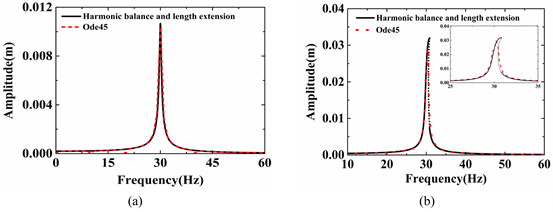Figure 3. Numerical solution and analytical solution (a) and (b)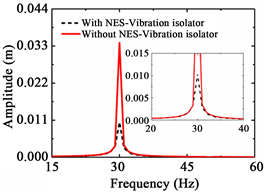Figure 4. The amplitude-frequency response curves with and without the NES-Vibration isolator system

3. 电压对比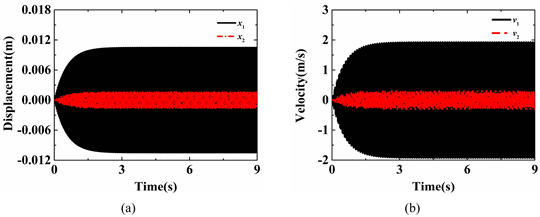Figure 5. Displacement relationship (a) and velocity relationship (b)

$\left\{\begin{array}{l}B\cong \underset{i=1}{\overset{8}{\sum }}{a}_{i}\mathrm{sin}\left({b}_{i}\chi +{c}_{i}\right)\\ \chi ={x}_{1}-{x}_{2}\end{array}$ (6)Table 2. Summation of sin function coefficients of fitted curve for the magnetic flux density, B

$V=\frac{BN2\pi r\left({\stackrel{˙}{x}}_{1}-{\stackrel{˙}{x}}_{2}\right)}{{R}_{\text{coil}}+{R}_{\text{load}}}{R}_{\text{load}}$ (7)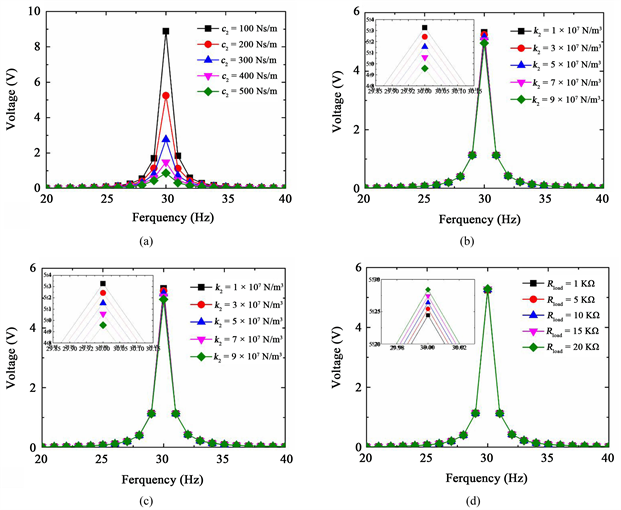Figure 6. Influence of system parameters on output voltage

4. 能量分析

$E\left(t\right)={T}_{\text{PS}}\left(t\right)+{V}_{\text{PS}}\left(t\right)+{W}_{\text{PS}}\left(t\right)+{T}_{\text{N-E}}\left(t\right)+{V}_{\text{N-E}}\left(t\right)+{W}_{\text{N-E}}\left(t\right)+{W}_{\text{ELE}}\left(t\right)$ (8)

${T}_{\text{PS}}\left(t\right)=\frac{1}{2}{m}_{1}{\stackrel{˙}{x}}_{1}^{2}\left(t\right)$ (9)

${V}_{\text{PS}}\left(t\right)=\frac{1}{2}{k}_{1}{\left[{x}_{1}\left(t\right)-u\left(t\right)\right]}^{2}$ (10)

${W}_{\text{PS}}={\int }_{0}^{t}{c}_{1}{\left[{\stackrel{˙}{x}}_{1}\left(t\right)-\stackrel{˙}{u}\left(t\right)\right]}^{2}\text{d}t;\text{\hspace{0.17em}}{W}_{\text{NES}}={\int }_{0}^{t}{c}_{2}{\left[{\stackrel{˙}{x}}_{1}\left(t\right)-{\stackrel{˙}{x}}_{2}\left(t\right)\right]}^{2}\text{d}t$ (11)

${T}_{\text{N-E}}\left(t\right)=\frac{1}{2}{m}_{2}{\stackrel{˙}{x}}_{2}^{2}\left(t\right)$ (12)

${V}_{\text{N-E}}\left(t\right)=\frac{1}{4}\left({k}_{2}+{k}_{m3}\right){\left[{x}_{1}\left(t\right)-{x}_{2}\left(t\right)\right]}^{4}+\frac{1}{2}\left({k}_{s}+{k}_{m1}\right){\left[{x}_{1}\left(t\right)-{x}_{2}\left(t\right)\right]}^{2}$ (13)

${W}_{\text{N-E}}\left(t\right)={\int }_{0}^{t}\left({c}_{2}+{c}_{a}+{c}_{m}\right){\left[{\stackrel{˙}{x}}_{1}\left(t\right)-{\stackrel{˙}{x}}_{2}\left(t\right)\right]}^{2}\text{d}t$ (14)

${W}_{\text{ELE}}\left(t\right)={\int }_{0}^{t}{\left(\frac{BNl\left({\stackrel{˙}{x}}_{1}\left(t\right)-{\stackrel{˙}{x}}_{2}\left(t\right)\right)}{{R}_{\text{coil}}+{R}_{\text{Load}}}\right)}^{2}{R}_{\text{Load}}\text{d}t$ (15)

${\eta }_{\text{PS}}=\frac{{T}_{\text{PS}}\left(t\right)+{V}_{\text{PS}}\left(t\right)+{W}_{\text{PS}}\left(t\right)}{E\left(t\right)}×100%$ (16)

NES-双向隔振器耦合系统吸收的能量占输入总能量的百分比表示如下。

${\eta }_{\text{N-E}}=\frac{{T}_{\text{N-E}}\left(t\right)+{V}_{\text{N-E}}\left(t\right)+{W}_{\text{N-E}}\left(t\right)+{W}_{\text{ELE}}\left(t\right)}{E\left(t\right)}×100%$ (17)

NES阻尼耗散掉的能量占输入总能量的百分比表示如下。

${\eta }_{\text{NES,W}}=\frac{{W}_{\text{NES}}\left(t\right)}{E\left(t\right)}×100%$ (18)

NES-双向隔振器耦合系统采集的能量占总能量的百分比表示如下。

${\eta }_{\text{ELE,W}}=\frac{{W}_{\text{ELE}}\left(t\right)}{E\left(t\right)}×100%$ (19)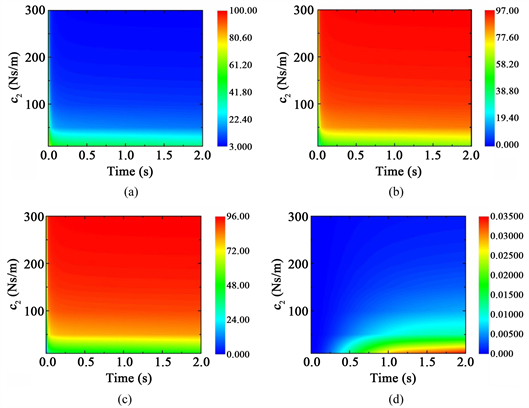Figure 7. The percentage of input energy absorbed by the primary system (a), the percentage of energy absorbed by the NES-dual-purpose isolator coupling system (b), the energy variation of the NES damping dissipation (c), and the percentage of energy collected by the coupled system (d), when the NES damper c2 variesFigure 8. The percentage of input energy absorbed by the primary system (a); the percentage of energy absorbed by the NES-dual-purpose isolator coupling system (b); the energy variation of the NES damping dissipation (c); and the percentage of energy collected by the coupled system (d); when the NES stiffness k2 varies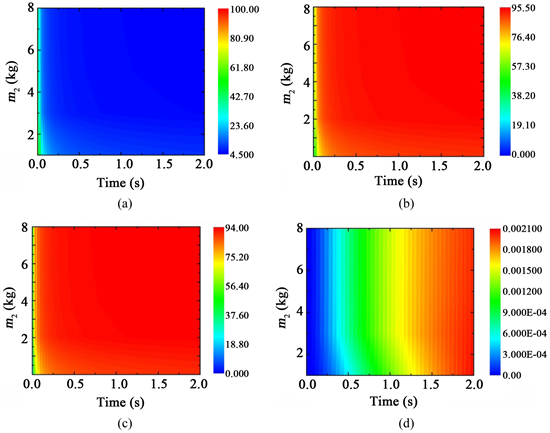Figure 9. The percentage of input energy absorbed by the primary system (a); the percentage of energy absorbed by the NES-dual-purpose isolator coupling system (b); the energy variation of the NES damping dissipation (c); and the percentage of energy collected by the coupled system (d); when the NES damper m2 varies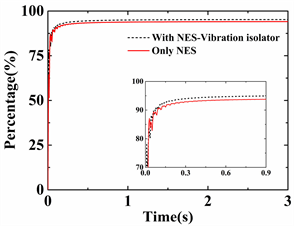Figure 10. Energy dissipation of NES coupling system with or without vibration isolator

5. 结论

1) 解析解与数值解的对比结果一致重合，证明了数值仿真结果的正确性。

2) 对比分析不同频率下系统的幅频响应，结果表明了NES-双向隔振器耦合系统具有更优良的减振性能。

3) 从能量转换的角度分析，耦合系统可以将系统能量转化为所需的电能输出，实现了对系统的能量采集，并且比传统NES系统具有更高的能量耗散率。

 Vakakis, A.F., Gendelman, O.V., Bergman, L.A., Mcfarland, D.M., Kerschen, G. and Lee, Y.S. (2009) Nonlinear Targeted Energy Transfer in Mechanical and Structural Systems II. Springer, Netherlands, 161-229.

 Gendelman, O.V. (2011) Targeted Energy Transfer in Systems with External and Self-Excitation. Proceedings of the Institution of Mechanical Engineers Part C: Journal of Mechanical Engineering Science, 225, 2007-2043.
https://doi.org/10.1177/0954406211413976

 Santos, M.P.S.D., Ferreira, J.A.F., Simões, J.A.O., Pascoal, R. and Furlani, E.P. (2016) Magnetic Levitation-Based Electromagnetic Energy Harvesting: A Semi-Analytical Non-Linear Model for Energy Transduction. Scientific Reports, 6.
https://doi.org/10.1038/srep18579

 Lu, Y., Capo-Chichi, M., Leprince-Wang, Y. and Basset, P. (2017) A Flexible Electrostatic Kinetic Energy Harvester Based on Electret Films of Electrospun Nanofibers. Smart Materials and Structures, 27.
https://doi.org/10.1088/1361-665X/aa87da

 Xiong, L., Tang, L., Liu, K., et al. (2018) Broadband Piezoelectric Vibration Energy Harvesting Using a Nonlinear Energy Sink. Journal of Physics D Applied Physics, 51.
https://doi.org/10.1088/1361-6463/aab9e3

 Chen, L.Q. and Jiang, W.A. (2015) Internal Resonance Energy Harvesting. Journal of Applied Mechanics, 82, 031004.
https://doi.org/10.1115/1.4029606

 Blokhina, E., Galayko, D., Basset, P., et al. (2013) Steady-State Oscillations in Resonant Electrostatic Vibration Energy Harvesters. IEEE Transactions on Circuits & Systems I Regular Papers, 60, 875-884.
https://doi.org/10.1109/TCSI.2012.2209295

 Lefeuvre, E., Badel, A., Richard, C., et al. (2006) A Comparison between Several Vibration-Powered Piezoelectric Generators for Standalone Systems. Sensors & Actuators a Physical, 126, 405-416.
https://doi.org/10.1016/j.sna.2005.10.043

 Mahdi, M.S.M. and Hamzeh, B. (2019) A Dual-Purpose Vibration Isolator Energy Harvester: Experiment and Model. Mechanical Systems and Signal Processing, 118, 360-376.
https://doi.org/10.1016/j.ymssp.2018.08.054

 Fang, Z.W., Zhang, Y.W., Li, X., et al. (2017) Integration of a Nonlinear Energy Sink and a Giant Magnetostrictive Energy Harvester. Journal of Sound & Vibration, 391, 35-49.
https://doi.org/10.1016/j.jsv.2016.12.019

Top